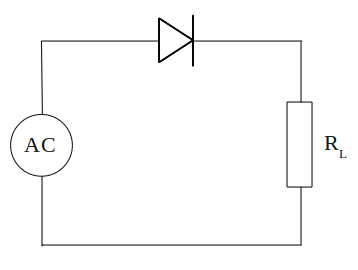# Half-wave rectifier circuit

One of most important use of diodes based on the ability of diode to conduct current in only one direction. When the diode is mounted on an alternating current or AC current, then the sine wave is converted into unidirectional wave or DC current.

Watch what happens to the circuit belowElectrical current is supplied to the circuit is an alternating current generated by a transformer. During the positive half cycle of AC, diodes are forward biased so current can flow. Current that flows through the diode to the load (RL) and back toward the transformer. Then the negative AC half cycle, diode does not conduct electric current, because given the reverse bias.

Waveforms of current through the load (RL) plot in the figure below.A circuit that is capable of converting AC voltage into DC is called as a rectifier circuit (rectifier). While the rectifier circuit as above, producing output current from the positive half cycle of input, we call it a half-wave rectifier circuit.
Next→
←Prev